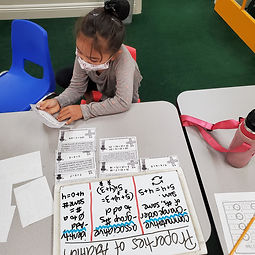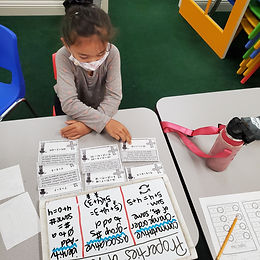## Ms. Raejean

### Target 1​

###### Lesson Type:

Continuation

Measurment

:

Time

Write and tell time to the nearest five minutes using analog and digital clocks.

###### 1:

Learn the meaning of time expressions such as half-past, quarter-past, and quarter-to.

###### 2:

Understand and use 24-hour notation (military time).

###### 3:

Link digital, analogy, a.m., p.m., and military time.

3rd

###### Vocabulary:

12-hour and 24-hour clocks, Quarter Past, Half Past, and Quarter To

Activities:

Students continued to practice telling time. Students wrote time using the 12-hour and 24-hour clocks. We also spent more time talking about the clock in fourths with ¼ or a quarter equaling 15 minutes. Students continued identifying time as a quarter past, half past, and quarter to.### Home Exploration

###### Guiding Questions:## Absent Students:

### Target 2

:

###### 1:

Identify the correct arithmetic processes based on the information presented in word problems.

###### 2:

Find the key words in word problems that indicate the correct arithmetic process to use.

###### 3:

Find and use the needed information in a word problem in order to solve.

###### 4:

Write an equation from a word problem with a variable included in the equation. Then solve for the variable.

3rd

###### Vocabulary:

Multi-step Problem, Equation, Variable, Unknown

Activities:

Students continued to read and make sense of multi-step word problems. Students identified the important information, determined what operations needed to be used, and wrote an equation with a variable to represent the unknown number. Then, students solved for the unknown.### Home Exploration

###### Guiding Questions:### Target 3

:

###### 1:

Identify the Associative Property of Addition.

###### 2:

Understand that the associative property is about grouping factors in an addition equation without changing the sum.

###### 3:

Understand that the associative property is about grouping factors in an addition equation without changing the sum.

###### 4:

Although computation often is necessary to solve in the order given, with addition, addends can be computed in an order that produces more “friendly” numbers.

3rd

###### Vocabulary:

Activities:### Home Exploration